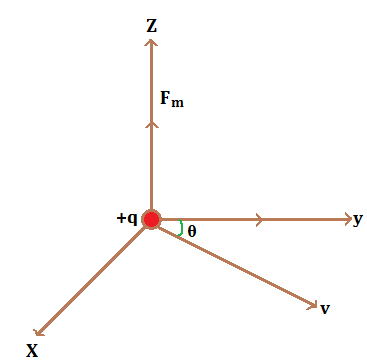# Force on Moving Charge in Magnetic FieldForce on moving charge in magnetic field

Let us consider a charge q moves with the velocity v through the magnetic field B as shown in figure. The magnetic force exerted by the charge is F.

Now, according to Lorentz, the force experienced by a moving charge in a uniform magnetic field is directly proportional to:

• Strength of magnetic field i.e., F ∝ B……(i)
• Magnitude of the charge i.e., F ∝ q…….(ii)
• Velocity of the charge i.e., F ∝ v……(iii)
• Angle between V and B i.e., F ∝ sinθ ……(iv)

Combining all the equations, we get:
F ∝ Bqvsinθ
F = k.Bqvsinθ                                                                                                                                                                                    Where k is a constant whose value is found to be 1.
∴ F = Bqvsinθ

Vectorically, $\vec F= q ( \vec v \times \vec B)$

Here, the direction of force is perpendicular to the plane of V and B. This force is also called as Lorentz magnetic force.

Case 1:
when θ =0°,
F = BqVsin0°=0
Case2:
when θ=90°
F = BqVsin90° =BqV
Case3:
When θ=180°
F = BqV180°
F = 0
Unit of B: The SI unit of magnetic field strength is Tesla.

We know,

$F= Bqvsin \theta$
$\text{or} B = \frac{F}{qv sin \theta }$
When charge (q) = 1 Colomb, velocity (v) = 1 metre per second and force = 1 N then,
$B = \frac{1}{1 \times 1 \times 1} = 1 \text{Tesla}$
So, the magnetic field strength at a point is 1 T if 1 charge is moving through 1 metre per second at right angle to the magnetic field and experiences 1 N magnetic force.

Do you like this article ? If yes then like otherwise dislike :